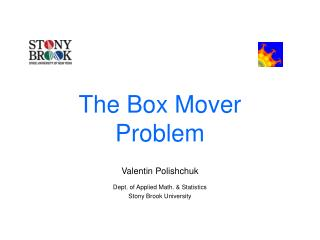DownloadDownload PresentationThe Box Mover Problem

# The Box Mover Problem

Télécharger la présentation## The Box Mover Problem

- - - - - - - - - - - - - - - - - - - - - - - - - - - E N D - - - - - - - - - - - - - - - - - - - - - - - - - - -
##### Presentation Transcript

1. The Box Mover Problem Valentin Polishchuk Dept. of Applied Math. & Statistics Stony Brook University

2. A Puzzle

3. A Puzzle • Pixeled domain

4. A Puzzle • Pixeled domain • Items • 1 per pixel

5. A Puzzle • Pixeled domain • Items • 1 per pixel • Agent (Robot) travels moves the items www.cs.utah.edu/~mflatt/

6. A Puzzle • Pixeled domain • Items • 1 per pixel • Agent (Robot) travels moves the items • initial position

7. A Puzzle • Pixeled domain • Items • 1 per pixel • Agent (Robot) travels moves the items • initial position • Rules/Constraints • What happens after a move • What is forbidden

8. A Puzzle (contd.) • Target configuration • Sink

9. A Puzzle (contd.) • Target configuration • Sink • Goal: • items • initial → target

10. A Puzzle (contd.) • Target configuration • Sink • Goal: • items • initial → target • robot • back

11. The Box Mover Problem

12. The Box Mover Problem • Domain – warehouse • Items – boxes

13. The Box Mover Problem • Domain – warehouse • Items – boxes • Agent (robot) – warehouse-keeper, pushing and/or pulling and/or lifting boxes

14. The Box Mover Problem • Domain – warehouse • Items – boxes • Agent (robot) – warehouse-keeper, pushing and/or pulling and/or lifting boxes • Rules • don’t step on boxes

15. The Box Mover Problem • Domain – warehouse • Items – boxes • Agent (robot) – warehouse-keeper, pushing and/or pulling and/or lifting boxes • Rules • don’t step on boxes • ≤k pushed • ≤p pulled • ≤l lifted BMP(k,p,l)

16. Versions of the Problem

17. Versions of the Problem • All boxes movable?

18. Versions of the Problem • All boxes movable? • Some fixed (rigid walls) BMP(k,p,l)-F • Robot’s path • No self-intersections BMP(k,p,l)-X • Boxes’ IDs #-BMP(k,p,l) E.g.: SOKOBAN = BMP(1,0,0)-F Push-k = BMP(k,0,0), Push-* = BMP(∞,0,0) BMP(∞, ∞, ∞) – Omnipotent Robot Problem

19. Versions of the Problem • All boxes movable? • Some fixed (rigid walls) BMP(k,p,l)-F • Robot’s path • No self-intersections BMP(k,p,l)-X • Boxes’ IDs #-BMP(k,p,l) E.g.: SOKOBAN = BMP(1,0,0)-F Push-k = BMP(k,0,0), Push-* = BMP(∞,0,0) BMP(∞, ∞, ∞) – Omnipotent Robot Problem

20. Versions of the Problem • All boxes movable? • Some fixed (rigid walls) BMP(k,p,l)-F • Robot’s path • No self-intersections BMP(k,p,l)-X • Boxes’ IDs #-BMP(k,p,l) E.g.: SOKOBAN = BMP(1,0,0)-F Push-k = BMP(k,0,0), Push-* = BMP(∞,0,0) BMP(∞, ∞, ∞) – Omnipotent Robot Problem

21. Versions of the Problem • All boxes movable? • Some fixed (rigid walls) BMP(k,p,l)-F • Robot’s path • No self-intersections BMP(k,p,l)-X • Boxes’ IDs #-BMP(k,p,l) E.g.: SOKOBAN = BMP(1,0,0)-F Push-k = BMP(k,0,0), Push-* = BMP(∞,0,0) BMP(∞, ∞, ∞) – Omnipotent Robot Problem

22. Previous work: Feasibility

23. Previous work: Feasibility • P? • monotone [Dhagat and O’Rourke,’92] • NP? • only 1 version (-F-X) [Demaine and Hoffmann,’01] • NP-hard, PSPACE-complete [Dhagat and O’Rourke,’92], [Dor and Zwick,’99], [Culberson,’98], [Demaine, Demaine and O'Rourke,’00], [Demaine, Demaine, Hoffmann and O'Rourke,’01], [Demaine, Hearn and Hoffmann,’02], [Hearn and Demaine,’02]

24. Previous work: Feasibility • P? • monotone [Dhagat and O’Rourke,’92] • NP? • only 1 version (-F-X) [Demaine and Hoffmann,’01] • NP-hard, PSPACE-complete [Dhagat and O’Rourke,’92], [Dor and Zwick,’99], [Culberson,’98], [Demaine, Demaine and O'Rourke,’00], [Demaine, Demaine, Hoffmann and O'Rourke,’01], [Demaine, Hearn and Hoffmann,’02], [Hearn and Demaine,’02]

25. “Reality”: Optimization

26. “Reality”: Optimization • “Infeasible!”– not acceptable • Upgrade robot, warehouse • Feasibility insured • Original SOKOBAN problem

27. “Reality”: Optimization • “Infeasible!”– not acceptable • Upgrade robot, warehouse • Feasibility insured • Original SOKOBAN problem • Optimization • cost = workload (pushes + pulls + lifts) • unloaded motion – no cost • [Sabey,’96]

28. Proving Hardness • All boxes movable, no walls, infinite plane • leakage is a problem • l = 0 • kp = 0 – a wall of thickness max(k, p)+1 is rigid • p = 0 – k+1-by-k+1 square is unmovable • k = 0 – p+1-by-p+1 square is unmovable Palliative, what if kp > 0 or l > 0 ? • Our hardness proof – wall thickness is constant

29. Making Puzzle “more tractable” • k ↑, p ↑, l ↑ ? • [Demaine, Demaine and O'Rourke,’00]: introducing powerful robot “makes it relatively easy to construct intractable puzzles” • constrain robot’s motion: heavier constructions • l>0 – none? • Mosaic Rearranging Problem (“flying” robot) is in P – solved by assignment • Limiting the robot’s capabilities • most problems (even the “more tractable” ones): • exact complexity unknown, some PSPACE-complete

30. Our Results • NP-hard for any (k, p, l) • including infinite values • Open BMP instances • in NP

31. Another Problem – Crossovers • [Demaine and Hoffman,‘01], [Demaine, Demaine, Hoffmann and O'Rourke,’01] contrasted their work to “all previous approaches of building circuits based on graphs, which seem to inherently require [problematic] crossings” • Our construction • no crossings • reduction from HC problem for planar graphs

32. [Culberson,’98]

33. The Reduction • HC in planar directed graphs • each node v: out(v) + in(v) = 3 [Plesnik,‘79]

34. BMP(1,0,0)-F Edges – corridors of width 1Nodes – T-intersections